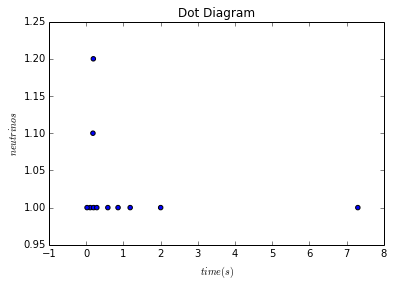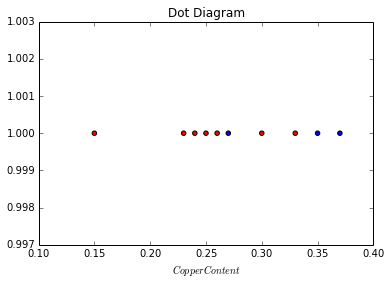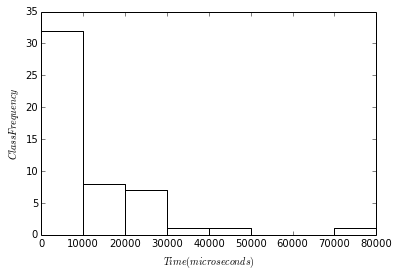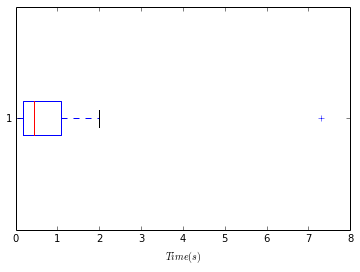Chapter 2: Organization and Description of Data¶

Example, page-13¶

In :
#Variable declaration
time=[0.107,0.196,0.021,0.283,0.179,0.854,0.58,0.19,7.3,1.18,2.0]  # Time in Seconds
neutrinos=[1,1,1,1,1.1,1,1,1.2,1,1,1]

#Calculation
from scipy import *
from pylab import *
%matplotlib inline

#Results
scatter(time,neutrinos)
title("Dot Diagram")
xlabel("$time(s)$")
ylabel("$neutrinos$")
Out:
<matplotlib.text.Text at 0x8170080>Example, page-14¶

In :
#Variable declaration
sample1 = [0.27,0.35,0.37]  # Copper content-1
sample2 = [0.23,0.15,0.25,0.24,0.30,0.33,0.26] # Copper content-2
Yvalue1 = [1,1,1]
Yvalue2 = [1,1,1,1,1,1,1]

#Calculation
from scipy import *
from pylab import *
%matplotlib inline

#Results
scatter(sample1,Yvalue1,c='b',marker='o')
scatter(sample2,Yvalue2,c='r',marker='o')

title("Dot Diagram")
xlabel("$Copper Content$")
Out:
<matplotlib.text.Text at 0x82c74e0>Example, Page-16¶

In :
#Variable declaration
l = [205,245,285,325,365,405]  # intervels
Marks = []

#Calculation
from scipy import *
from pylab import *

for i in range(0,5):
Marks.append((l[i]+l[i+1])/2)

#Results
print "Class Average: "
for each in Marks:
print each," "
print "Class Interval: ",l-l
Class Average:
225
265
305
345
385
Class Interval:  40

Example, Page-16¶

In :
#Variable declaration
l = [205,245,285,325,365,405]  # intervels
frequency = [3,11,23,9,4]
cal = []
sum1 = 0
#Calculation
from scipy import *
from pylab import *

for i in range(1,6):
for j in range(0,i):
sum1 = sum1 + frequency[j]
cal.append(sum1)
sum1 = 0

#Results
print "Cumulative Frequency: "
for i in range(0,5):
print "(",l[i],",",l[i+1],"]","  ",cal[i]
Cumulative Frequency:
( 205 , 245 ]    3
( 245 , 285 ]    14
( 285 , 325 ]    37
( 325 , 365 ]    46
( 365 , 405 ]    50

Example, Page-19¶

In :
#Variable declaration
l = [2808, 4201, 3848, 9112, 2082, 5913, 1620, 6719, 21657,
3072, 2949, 11768, 4731, 14211, 1583, 9853, 78811, 6655,
1803, 7012, 1892, 4227, 6583, 15147, 4740, 8528, 10563,
43003, 16723, 2613, 26463, 34867, 4191, 4030, 2472, 28840,
24487, 14001, 15241, 1643, 5732, 5419, 28608, 2487, 995,
3116, 29508, 11440, 28336, 3440]
height = [0,0,0,0,0,0,0,0,0,0,0]
X = [0,10000,20000,30000,40000,50000,60000,70000,80000,90000,100000]

#Calculation
from scipy import *
from pylab import *
%matplotlib inline

for each in l:
height[each/10000 ] = height[each/10000 ] + 1

#Results
bar(X,height,width=10000,fill=False)
xlabel("$Time(microseconds)$")
ylabel("$ClassFrequency$")
Out:
<matplotlib.text.Text at 0x988d588>Example, page-25¶

In :
#Variable declaration
l=[15,14,2,27,13]        #list of numbers of meals claimed

#Calculation
from scipy import *
Median=median(l)         #median of all entries
Mean=round(mean(l),2)    #mean of all entries

#Results
print "mean: ",Mean,"meals"
print "median: ",int(Median),"meals"
mean:  14.2 meals
median:  14 meals

Example, page-25¶

In :
#Variable declaration
l=[11,9,17,19,4,15]        #list of numbers of e-mail received for six day

#Calculation
from scipy import *
Median=median(l)         #median of all entries
Mean=round(mean(l),2)    #mean of all entries

#Results
print "mean: ",Mean,"requests"
print "median: ",int(Median),"requests"
mean:  12.5 requests
median:  13 requests

Example, page-27¶

In :
#Variable declaration
l=[0.6,1.2,0.9,1.0,0.6,0.8]      #list of delay times
k=0

#Calculation
from scipy import *
from pylab import *
Mean=round(mean(l),4)
for each in l:
k+=(each-Mean)**2

ssquare=round(k/(len(l)-1),3)   # Sample Variance (in minute square)

#Results
print "Sample Variance: ",ssquare,"(minute square)"
Sample Variance:  0.055 (minute square)

Example, page-28¶

In :
#Variable declaration
l=[0.6,1.2,0.9,1.0,0.6,0.8]      #list of delay times
k=0

#Calculation
from scipy import *
from pylab import *
Mean=round(mean(l),4)
for each in l:
k+=(each-Mean)**2

s=sqrt(round(k/(len(l)-1),3))   # Standard deviation (in minute)
s=round(s,2)

#Results
print "Standard deviation: ",s,"minute"
Standard deviation:  0.23 minute

Example, page-29¶

In :
#Variable declaration
stddev1 = 0.0152    #standard deviation for ball bearing (in mm)
mean1 = 3.92        #mean for ball bearing (in mm)
stddev2 = 0.0086    #standard deviation for spring (in inch)
mean2 = 1.54        #mean for spring (in inch)

#Calculation
from scipy import *
from pylab import *
cof_var1 = round((stddev1/mean1)*100,3)   # coff of variation for ball bearing in %
cof_var2 = round((stddev2/mean2)*100,3)   # coff of variation for spring in %

#Results
if cof_var1<cof_var2:
print "First instrument is more precise"
elif cof_var1>cof_var2:
print "Second instrument is more precise"
else:
print "both instruments are equal precise"

First instrument is more precise

Example, page-30¶

In :
#Variable declaration
l = [221, 234, 245, 253, 265, 266, 271, 272, 274, 276,
276, 276, 278, 284, 289, 290, 290, 292, 292, 296,
297, 298, 300, 303, 304, 305, 305, 308, 308, 309,
310, 311, 312, 314, 315, 315, 323, 330, 333, 336,
337, 338, 343, 346, 355, 364, 366, 373, 390, 391]

#Calculation
from scipy import *
from pylab import *

np = len(l)*0.25              # np-losition in list l[],for first quartile p=1/4
Q1 = l                    # as np=12.5,so we round up to 13th
np = len(l)*0.5               #for second quartile p=1/2
np=int(np)
Q2 = (l[np-1] + l[np])*0.5    # Average of 25th and 26th
np = len(l)*0.75              #for third quartile p=3/4
Q3=l                      # round up to 38th
np = len(l)*0.93              #for 93rd percentile p=0.93
Q93=l                     # round up to 47th

#Results
print "First quartile Q1: ",Q1,"nm"
print "Second quartile Q2: ",Q2,"nm"
print "Third quartile Q3: ",Q3,"nm"
print "93rd quartile Q93: ",Q93,"nm"
First quartile Q1:  278 nm
Second quartile Q2:  304.5 nm
Third quartile Q3:  330 nm
93rd quartile Q93:  366 nm

Example, page-31¶

In :
#Variable declaration
l = [221, 234, 245, 253, 265, 266, 271, 272, 274, 276,
276, 276, 278, 284, 289, 290, 290, 292, 292, 296,
297, 298, 300, 303, 304, 305, 305, 308, 308, 309,
310, 311, 312, 314, 315, 315, 323, 330, 333, 336,
337, 338, 343, 346, 355, 364, 366, 373, 390, 391]           #list of all height entries

#Calculation
from scipy import *
from pylab import *

np = len(l)*0.25    # np-losition in list l[],for first quartile p=1/4
Q1 = l  # as np=12.5,so we round up to 13th
np = len(l)*0.5    #for second quartile p=1/2
np = int(np)
Q2 = (l[np-1] + l[np])*0.5   # Average of 25th and 26th
np = len(l)*0.75    #for third quartile p=3/4
Q3 = l    # round up to 38th
rng = max(l)-min(l)   #range of height
int_rng = Q3-Q1    #interquartile range of height

#Results
print "range : ",rng,"nm"
print "interquartile range : ",int_rng,"nm"
range :  170 nm
interquartile range :  52 nm

Example, Page-32¶

In :
#Variable declaration
x = [0.021, 0.107, 0.179, 0.190, 0.283, 0.580, 0.854, 1.18, 2.00, 7.30]

#Calculation
from scipy import *
from pylab import *
import matplotlib.pyplot as plt
%matplotlib inline

plt.boxplot(x,vert=False)
xlabel("$Time(s)$")
Out:
<matplotlib.text.Text at 0x9b1e1d0>Example, Page-34¶

In :
#Variable declaration
l = [19.7, 21.5, 22.5, 22.2, 22.6,
21.9, 20.5, 19.3, 19.9, 21.7,
22.8, 23.2, 21.4, 20.8, 19.4,
22.0, 23.0, 21.1, 20.9, 21.3]
sum1 = 0.0
#Calculation
from scipy import *
from pylab import *

Mean=sum(l)/len(l)

for each in l:
sum1 = sum1 + each*each
variance = (sum1 - (pow(sum(l),2.0)/len(l)))/(len(l)-1)   # variance
variance= round(variance,3)

#Results
print "mean: ",Mean,"mpg"
print "variance: ",variance
mean:  21.385 mpg
variance:  1.412

Example, page-35¶

In :
#Variable declaration
x = array([[225,3],[265,11],[305,23],[345,9],[385,4]])
temp1 = 0
temp2 = 0

#Calculation
from scipy import *
from pylab import *
from numpy import *

for i in range(0,5):
temp1 = temp1 + x[i]*x[i]

Mean = temp1/sum(x[0:5,1])    # mean=sum(x(i)*f(i))/sum(f(i)  class average

for i in range(0,5):
temp2 = temp2 + x[i]*x[i]*x[i]

variance = (temp2 - (temp1**2) / sum(x[0:5,1])) / float(sum(x[0:5,1])-1)   # variance
variance=round(variance,2)
std_dev=round(sqrt(variance),1)             # standard deviation

#Results
print "mean: ",Mean
print "variance: ",variance
print "standard deviation: ",std_dev
mean:  305
variance:  1567.35
standard deviation:  39.6